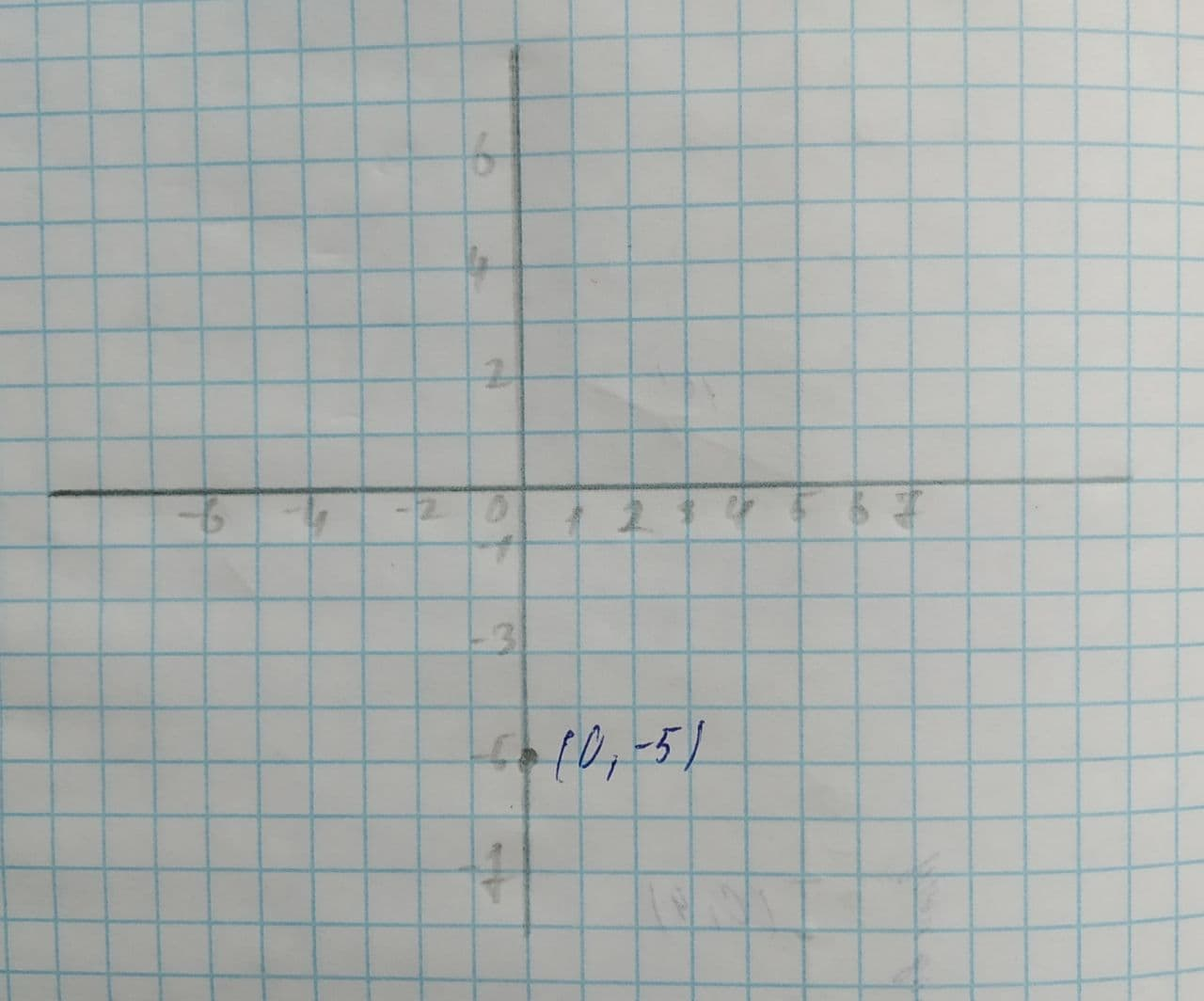Question# Plot a complex number -5i and graph. Specify the absolute value of the number.

ANSWEREDPlot a complex number graph. Specify the absolute value of the number.
-5i2021-07-28

Step 1: Consider the provided complex expression,
-5i
The graph of the complex number is shown below,Step 2: The absolute value of the expression is calculated below,
$$\displaystyle{\left|-{5}{i}\right|}=\sqrt{{{\left(-{5}\right)}^{{{2}}}}}$$
$$\displaystyle=\sqrt{{{25}}}$$
$$\displaystyle=\pm{5}$$
Therefore, the absolute value of the function is $$\displaystyle\pm{5}$$.